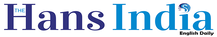Top

# Tips to crack Eamcet MathsHighlights

## With Eamcet around the corner, here are a few tips to score well in Mathematics, the most important subject with a weightage of 80 marks as against...

With Eamcet around the corner, here are a few tips to score well in Mathematics, the most important subject with a weightage of 80 marks as against Physics, Chemistry (each carries 40 marks)1. There are four main segments- Algebra, Trignometry, Calculus and Co-ordinate Geometry. All the four segments share equal number of questions and marks. So all the four segments must be thoroughly practiced.

2. Remember all the formulas of all four segments.

3. Matrices, functions, complex numbers, quadratic equations are the easiest chapters, so practice them at the end.

4. Calculus (integrations and differentiations), vector algebra and co-ordinate geometry are scoring subjects. Expect approximately 46 questions from those chapters.

5. The most important tip: Most of the students commit a blunder in the examination by solving the problems one by one before going through the paper thoroughly. There are few standard questions which need not to be solved, but can be answered directly. Such questions are to be solved first to save time for the other subjects too.